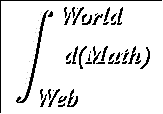# The Squeeze Theorem Applied to Useful Trig Limits

Suggested Prerequesites: The Squeeze Theorem, An Introduction to Trig

There are several useful trigonometric limits that are necessary for evaluating the derivatives of trigonometric functions. Let's start by stating some (hopefully) obvious limits:

#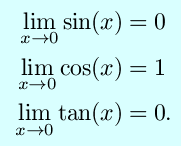Since each of the above functions is continuous at x = 0, the value of the limit at x = 0 is the value of the function at x = 0; this follows from the definition of limits.

In order to evaluate the derivatives of sine and cosine we need to evaluate

#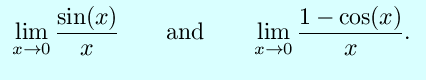In order to find these limits, we will need the following theorem of geometry: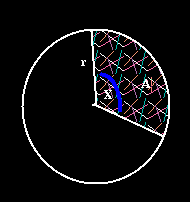If x is the measure of the central angle of a circle of radius r, then the area A of the sector determined by x is
A = r2x/2

Let's start by looking at

#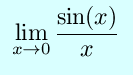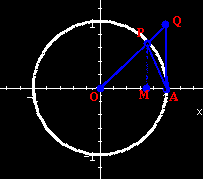If

#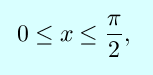we have the situation in the figure to the left. Assume the circle is a unit circle, parameterized by x = cos t, y = sin t
(for the rest of this page, the arguments of the trig functions will be denoted by t instead of x, in an attempt to reduce confusion with the cartesian coordinate).If A1 is the area of the triangle AOP, A2 is the area of the circular sector AOP, and A3 is the area of the triangle AOQ,

A1 < A2 < A3.

The area of a triangle is equal to one-half of the product of the base times the height. Using this well-known result, and the above theorem for the area of a sector of a circle (with t as the central angle), we obtain:

It follows that

and hence

#which is equivalent to

#These three functions are easily plotted; the yellow line is the plot of the constant function 1, the magenta is the cosine, and the red is sin(t)/t.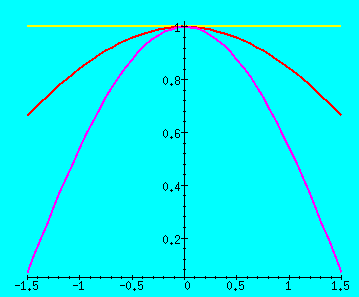From the Squeeze Theorem, it follows that

To find

#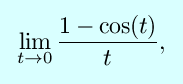we do some algebraic manipulations and trigonometric reductions:

#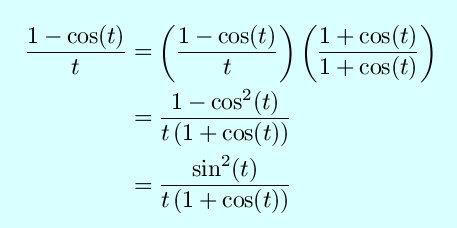Therefore, it follows that